Question

A multiple-choice test consists of 23 questions with possible answers of a, b, c, d. Estimate the probability that with random guessing, the number of correct answers is at least 10.

Solution :

A binomial with ,

n = 23

p = 0.25

P(X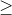10)

= 1 - BINOM.DIST(9 , 23 , 0.25 , TRUE)

= 0.0408

Probability = 0.0408

#### Earn Coins

Coins can be redeemed for fabulous gifts.

Similar Homework Help Questions
• ### (1 point) A multiple-choice test consists of 22 questions with possible answers of a, b, c,...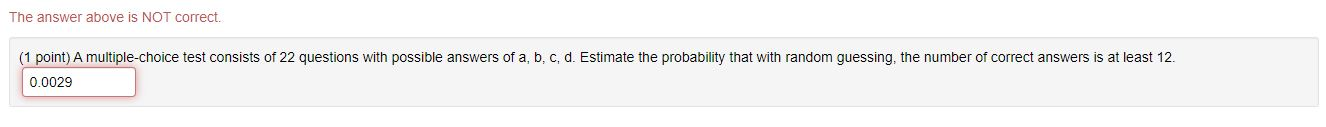(1 point) A multiple-choice test consists of 22 questions with possible answers of a, b, c, d. Estimate the probability that with random guessing, the number of correct answers is at least 12. The answer above is NOT correct. (1 point) A multiple-choice test consists of 22 questions with possible answers of a, b, c, d. Estimate the probability that with random guessing, the number of correct answers is at least 12. 0.0029

• ### Q. 27 A multiple choice test consists of 25 questions with possible answers a, b, c,...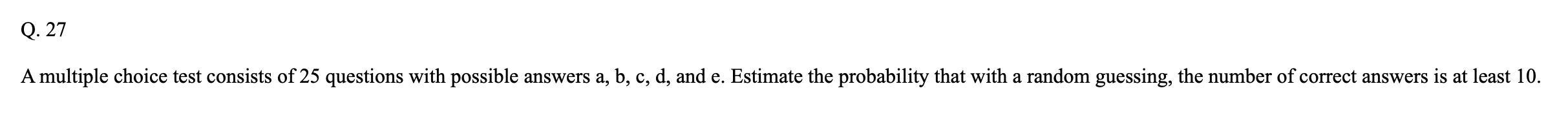Q. 27 A multiple choice test consists of 25 questions with possible answers a, b, c, d, and e. Estimate the probability that with a random guessing, the number of correct answers is at least 10.

• ### A multiple choice test consists of 10 questions each with four choices (a,b,c,d) for each question....

A multiple choice test consists of 10 questions each with four choices (a,b,c,d) for each question. If a student must get at least 7 correct to pass the test, what is the probability that a student could pass the exam simply by guessing? Find your answer to 4 decimal places.

• ### A multiple choice test consists of 60 questions. each question has 4 possible answers of which...

A multiple choice test consists of 60 questions. each question has 4 possible answers of which one is correct. If all answers are random guesses, estimate the probability that of 140 randomly selected seeds, exactly 100 will germinate

• ### A quiz consists of 10 multiple-choice questions, each with 5 possible answers. For someone who makes...

A quiz consists of 10 multiple-choice questions, each with 5 possible answers. For someone who makes random guesses for all of the answers, find the probability they get 6 or more questions correct. Use at least 5 decimals of accuracy in your answer. Answer=

• ### A multiple-choice examination consists of 75 questions, each having possible choices a, b, c, and d....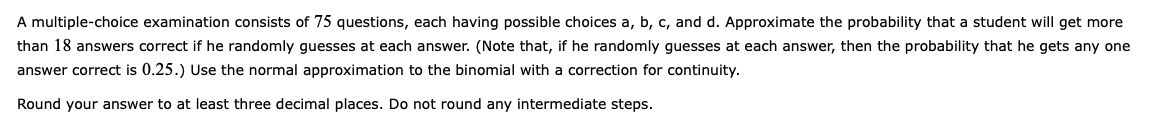A multiple-choice examination consists of 75 questions, each having possible choices a, b, c, and d. Approximate the probability that a student will get more than 18 answers correct if he randomly guesses at each answer. (Note that, if he randomly guesses at each answer, then the probability that he gets any one answer correct is 0.25.) Use the normal approximation to the binomial with a correction for continuity. Round your answer to at least three decimal places. Do not...

• ### A student answers all 48 questions on a multiple-choice test by guessing. Each question has four...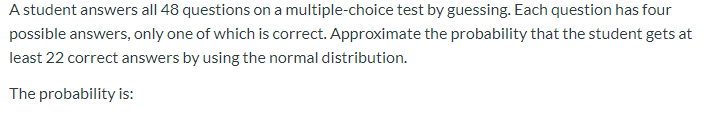A student answers all 48 questions on a multiple-choice test by guessing. Each question has four possible answers, only one of which is correct. Approximate the probability that the student gets at least 22 correct answers by using the normal distribution. The probability is:

• ### You are taking a multiple-choice quiz that consists of 3 questions

You are taking a multiple-choice quiz that consists of 3 questions. Each question has 4 possible answers, only one of which is correct. To complete the quiz, you randomly guess the answer to each question. Find the probability of guessing (A) exactly 2 answers correctly, (B) at least 2 answers correctly, or (C) less than 2 answers correctly.

• ### A test consists of 60 multiple choice questions, each with five possible answers, only one of...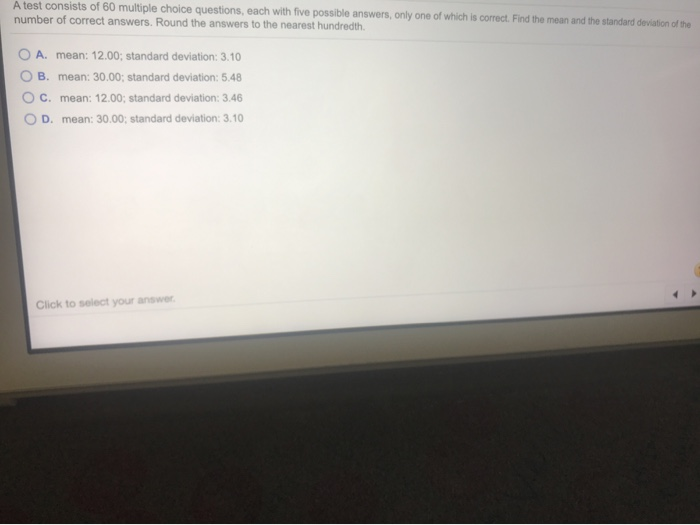A test consists of 60 multiple choice questions, each with five possible answers, only one of which is correct. Find the mean and the standard deviation of the number of correct answers. Round the answers to the nearest hundredth. ООО A. mean: 12.00; standard deviation: 3.10 B. mean: 30.00; standard deviation: 5:48 c. mean: 12.00; standard deviation: 3.46 D. mean: 30.00; standard deviation: 3.10 Click to select your answer.

• ### A student takes a quiz consisting of 10 multiple choice questions. Each question has 5 possible...

A student takes a quiz consisting of 10 multiple choice questions. Each question has 5 possible answers. If a student is guessing the answers at random, and theanswers to different questions are independent, find the probability of giving at least five correct answers.